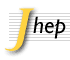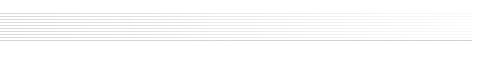## Keyword List

 Note The keywords are organized in sections. The section name is highlighted in grey followed by the list of individual keywords belonging to that section.

 Group: Experimental
Collider Physics Underground and Large Array Physics
• e+-e- Experiments
• Heavy Ion Experiments
• Lepton-Nucleon Scattering (experiments)
• Dark Matter and Double Beta Decay (experiments)
• Gamma and Cosmic Rays (experiments)
• Neutrino Detectors and Telescopes (experiments)
Non collider experiments with beams Optional keywords (supplementary)
• Fixed target experiments
• Other experiments
• Jet substructure
• B physics
• Beyond Standard Model
• Branching fraction
• Charm physics
• chiral b'
• CKM angle alpha
• CKM angle beta
• CKM angle gamma
• Collective flow
• CP violation
• Dark matter
• Diffraction
• Electroweak interaction
• Event-by-event fluctuation
• Exotics
• FCNC Interaction
• Flavor physics
• Flavour Changing Neutral Currents
• Forward physics
• four-top-production
• Global features
• Hard scattering
• harmonic flow
• Heavy quark production
• Heavy-ion collision
• Higgs physics
• Jet physics
• Jets
• Lepton production
• Minimum bias
• Oscillation
• Particle and resonance production
• Particle correlations and fluctuations
• Phase transitions
• Photon production
• Polarization
• proton-proton scattering
• QCD
• Quark deconfinement
• Quark gluon plasma
• Quarkonium
• Rare decay
• Relativistic heavy ion physics
• same charge top pair
• Spectroscopy
• Supersymmetry
• Tau Physics
• Top physics
• top squark
• Unfolding
• vector-like quarks
 Group: Theoretical
Quantum Field Theory Gauge Field Theories
• BRST Quantization
• Conformal Field Theory
• Effective Field Theories
• Field Theories in Higher Dimensions
• Field Theories in Lower Dimensions
• Large Extra Dimensions
• Lattice Quantum Field Theory
• Nonperturbative Effects
• Renormalization Group
• Renormalization Regularization and Renormalons
• Sigma Models
• Solitons Monopoles and Instantons
• Supersymmetric Gauge Theory
• Topological Field Theories
• 1/N Expansion
• Anyons
• Chern-Simons Theories
• Confinement
• Duality in Gauge Field Theories
• Scattering Amplitudes
• Spontaneous Symmetry Breaking
• Topological States of Matter
• Wilson, 't Hooft and Polyakov loops
Symmetries String and Brane Theory
• Anomalies in Field and String Theories
• Conformal and W Symmetry
• Discrete Symmetries
• Gauge Symmetry
• Global Symmetries
• Higher Spin Symmetry
• Space-Time Symmetries
• Black Holes in String Theory
• Bosonic Strings
• Brane Dynamics in Gauge Theories
• Conformal Field Models in String Theory
• D-branes
• F-Theory
• Flux compactifications
• Gauge-gravity correspondence
• Holography and condensed matter physics (AdS/CMT)
• Holography and quark-gluon plasmas
• Long strings
• M-Theory
• M(atrix) Theories
• p-branes
• String Duality
• String Field Theory
• String theory and cosmic strings
• Superstring Vacua
• Superstrings and Heterotic Strings
• Tachyon Condensation
• Topological Strings
General Relativity and Gravitation Supersymmetry
• 2D Gravity
• Black Holes
• Classical Theories of Gravity
• Higher Spin Gravity
• Lattice Models of Gravity
• Models of Quantum Gravity
• Spacetime Singularities
• Extended Supersymmetry
• Supergravity Models
• Superspaces
• Supersymmetric Effective Theories
• Supersymmetry and Duality
• Supersymmetry Breaking
Mathematical Methods of Physics Mostly Solvable Models
• Differential and Algebraic Geometry
• Integrable Hierarchies
• Non-Commutative Geometry
• Quantum Groups
• Stochastic Processes
• Integrable Field Theories
• Lattice Integrable Models
• Matrix Models
Astroparticles Statistical Field Theories
• Cosmology of Theories beyond the SM
• Solar and Atmospheric Neutrinos
• Thermal Field Theory
• Bethe Ansatz
• Boundary Quantum Field Theory
• Quantum Dissipative Systems
• Random Systems
Mostly Weak Interactions Mostly Strong Interactions
• Beyond Standard Model
• Compactification and String Models
• CP violation
• GUT
• Heavy Quark Physics
• Higgs Physics
• Kaon Physics
• Neutrino Physics
• Precision QED
• Quark Masses and SM Parameters
• Supersymmetric Standard Model
• Technicolor and Composite Models
• Chiral Lagrangians
• Lattice QCD
• Perturbative QCD
• Phase Diagram of QCD
• Quark-Gluon Plasma
• Resummation
 Group: Phenomenological
Quantum Field Theory (phenomenology) Strings and branes
• Lattice field theory simulation
• Non-perturbative renormalization
• Phenomenology of Field Theories in Higher Dimensions
• Phenomenology of Large extra dimensions
• Strings and branes phenomenology
Phenomenological Aspects of Supersymmetry Mostly Strong Interactions (phenomenology)
• Supersymmetry Phenomenology
• Deep Inelastic Scattering (Phenomenology)
• Heavy Ion Phenomenology
• Jets
• NLO Computations
• Phenomenological Models
• QCD Phenomenology